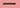# Conditional Rendering in SvelteIn this tutorial, we are going to learn about how to render elements conditionally in svelte.js.

## What is conditional rendering?

Conditional rendering means to show or hide elements from the dom based on the particular condition.

Svelte has a special syntax to render elements conditionally.

``````{#if isActive}
<h1>Im active</h1>
{/if}``````

Note: # indicates the block opening and `/` indicates block closing.

Let’s see an example.

Conditional.svelte
``````<script>
let isActive = true;</script>

<div>
{#if isActive}         <h1>Im active</h1>      {/if}  </div>``````

In the above code, we only render the `h1` element into the dom if `isActive` property is true.

## Else block

We can also combine `if` block with `else` block.

Conditional.svelte
``````<script>
let isActive = true;</script>

<div>
{#if isActive}
<h1>You can see me</h1>
{:else}         <h2>You can't see me</h2>      {/if}
</div>``````

## Else if block

We can also further chain it with else if block.

Conditional.svelte
``````<script>
let isActive = true;</script>

<div>
{#if isActive === true}
<h1>You can see me</h1>
{:else if isActive === 4}    <h1>You can see me only if isActive is 4</h1>  {:else}
<h2>You can't see me</h2>
{/if}
</div>``````

In the above code, we only render `else if` block `h1` element if `isActive === 4`.